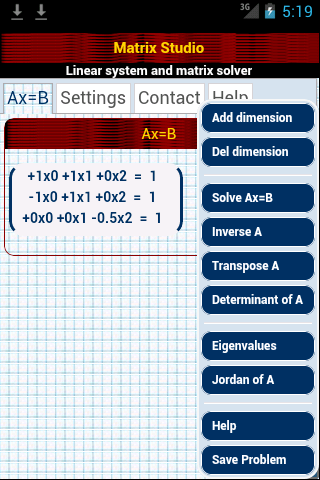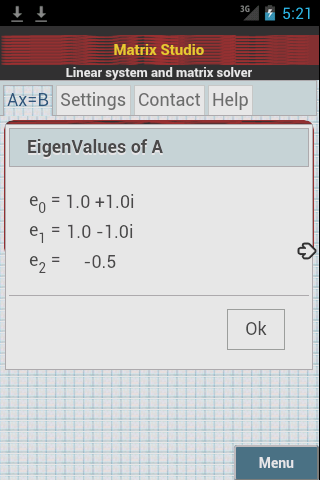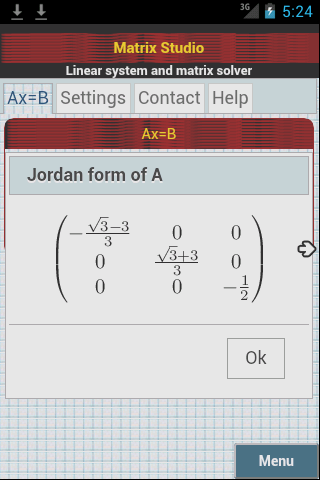# Matrix Studio: The Simplyest android matrix calculator

### Basic concepts

Matrix Studio is an application for Android devices to solve Ax=B linear equation systems and make several matrix operations such as inverse, determinant, transpose, eigenvalues, Jordan form, etc ...Screen for input matrix and menu sowing Screen sample for eigenvalues calculationsScreen on simply keyboard Example of Jordan cannonical form output calculations

## Was useful? want add anything?

Post here

### Post from other users

Post here